Inverse FunctionsCustom SearchINVERSE FUNCTIONS Theorem 7. The derivative of an inverse function is equal to the reciprocal of the derivative of the direct function. In the equations to this point, x has been the independent variable and y has been the dependent variable. The equations have been in a form such as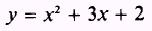Suppose that we have a function likeand we wish to find the derivative. Notice that if we solve for y in terms of x, using the quadratic formula, we get the more com�plicated function: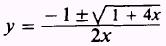if we call this function the direct function, thenis the inverse function. To determine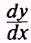from the inverse function is easy. EXAMPLE: Find the derivativeof the function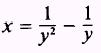SOLUTION.The derivative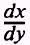isThe reciprocal ofis the derivative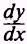of the direct function, and we find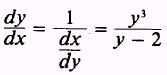EXAMPLE: Find the derivativeof the function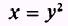SOL UTION.- Findto beThen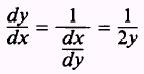PRACTICE PROBLEMS: Find the derivative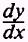of the following functions: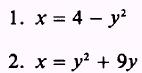ANSWERS:Integrated Publishing, Inc. - A (SDVOSB) Service Disabled Veteran Owned Small Business